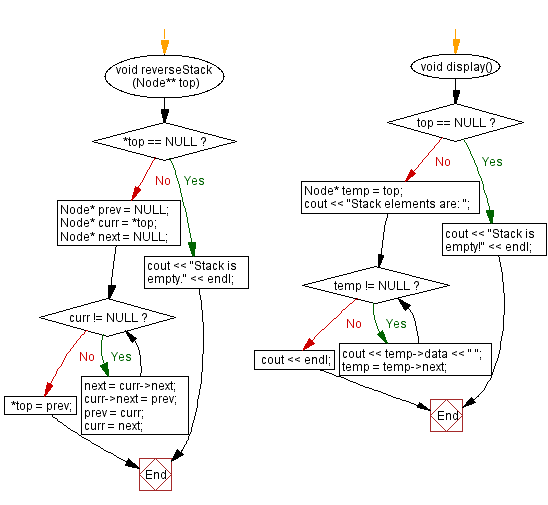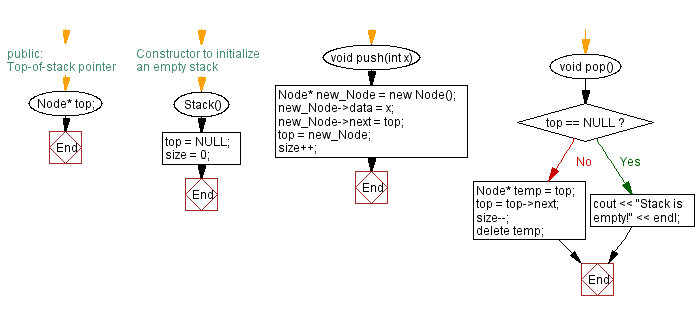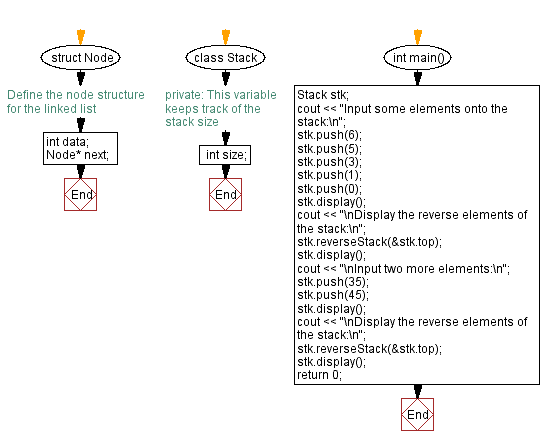﻿ C++ Reverse the elements of a stack (using a linked list)

# C++ Stack Exercises: Reverse the elements of a stack (using a linked list)

## C++ Stack: Exercise-18 with Solution

Write a C++ program to reverse the elements of a stack (using a linked list).

Test Data:
Input some elements onto the stack:
Stack elements are: 0 1 3 5 6
Display the reverse elements of the stack:
Stack elements are: 6 5 3 1 0

Sample Solution:

C++ Code:

``````#include <iostream>

using namespace std;

// Define the node structure for the linked list
struct Node {
int data;
Node* next;
};

class Stack {
private:

//This variable keeps track of the stack size
int size;

public:
// Top-of-stack pointer
Node* top;
// Constructor to initialize an empty stack
Stack() {
top = NULL;
size = 0;
}

void push(int x) {
Node* new_Node = new Node();
new_Node->data = x;
new_Node->next = top;
top = new_Node;
size++;
}
void pop() {
if (top == NULL) {
cout << "Stack is empty!" << endl;
return;
}
Node* temp = top;
top = top->next;
size--;
delete temp;
}

void reverseStack(Node** top) {
if (*top == NULL) {
cout << "Stack is empty." << endl;
return;
}

Node* prev = NULL;
Node* curr = *top;
Node* next = NULL;

while (curr != NULL) {
next = curr->next;
curr->next = prev;
prev = curr;
curr = next;
}

*top = prev;
}
void display() {
if (top == NULL) {
cout << "Stack is empty!" << endl;
return;
}
Node* temp = top;
cout << "Stack elements are: ";
while (temp != NULL) {
cout << temp->data << " ";
temp = temp->next;
}
cout << endl;
}
};

int main() {
Stack stk;
cout << "Input some elements onto the stack:\n";
stk.push(6);
stk.push(5);
stk.push(3);
stk.push(1);
stk.push(0);
stk.display();
cout << "\nDisplay the reverse elements of the stack:\n";
stk.reverseStack(&stk.top);
stk.display();
cout << "\nInput two more elements:\n";
stk.push(35);
stk.push(45);
stk.display();
cout << "\nDisplay the reverse elements of the stack:\n";
stk.reverseStack(&stk.top);
stk.display();
return 0;
}
``````

Sample Output:

```Input some elements onto the stack:
Stack elements are: 0 1 3 5 6

Display the reverse elements of the stack:
Stack elements are: 6 5 3 1 0

Input two more elements:
Stack elements are: 45 35 6 5 3 1 0

Display the reverse elements of the stack:
Stack elements are: 0 1 3 5 6 35 45
```

Flowchart:CPP Code Editor:

Previous C++ Exercise: Check the size and empty status of a stack (linked list).
Next C++ Exercise: Sort the elements of a stack (using a linked list).

What is the difficulty level of this exercise?

﻿

## C++ Programming: Tips of the Day

Can you remove elements from a std::list while iterating through it?

You have to increment the iterator first (with i++) and then remove the previous element (e.g., by using the returned value from i++). You can change the code to a while loop like so:

```std::list<item*>::iteratori = items.begin();
while (i != items.end())
{
boolisActive = (*i)->update();
if (!isActive)
{
items.erase(i++);  // alternatively, i = items.erase(i);
}
else
{
other_code_involving(*i);
++i;
}
}
```

Ref : https://bit.ly/3smQY4u